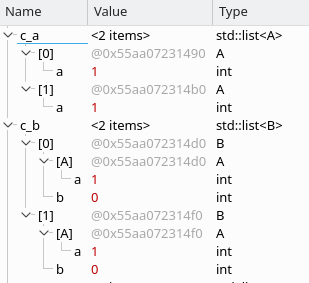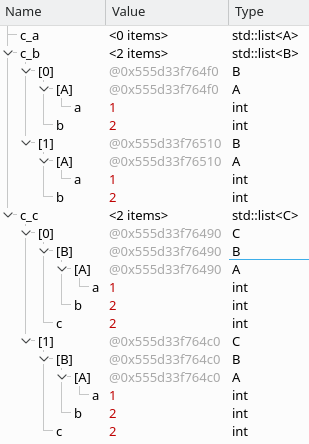# C++ 容器 父类转子类 子类转父类

class A {
public:
int a = 1;
};

class B : A {
public:
int b = 2;
};

class C : B {
public:
int c = 2;
};


int main()
{
list<A> c_a;
list<B> c_b;
list<C> c_c;
for (int i = 0; i < 2; ++i) {
c_a.push_back(A());
}
c_b = *reinterpret_cast <list<B> *> (&c_a);
return 0;
}int main()
{
list<A> c_a;
list<B> c_b;
list<C> c_c;
for (int i = 0; i < 2; ++i) {
c_c.push_back(C());
}
c_b = *reinterpret_cast <list<B> *> (&c_c);
return 0;
}## 《C++ 容器 父类转子类 子类转父类》有2个想法

1.COCO

1.admin 文章作者